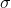# Question A point charge q = 3.80 nC is 8.00 cm from a thin, flat, infinite sheet that has a uniform surface charge density. If the total electric field is zero at a point halfway between the point charge and the sheet, what is the surface charge density of the sheet? ==